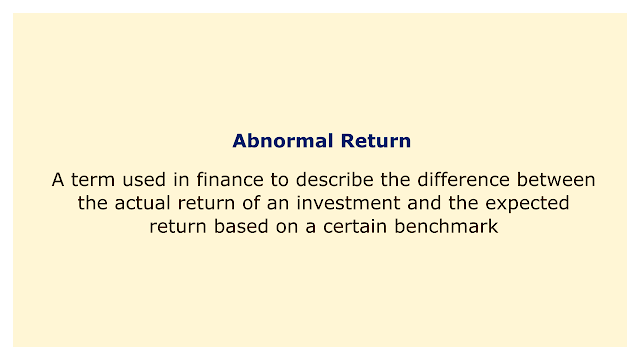# Abnormal ReturnImage: Moneybestpal.com

### Abnormal return is a term used in finance to describe the difference between the actual return of an investment and the expected return based on a certain benchmark or model. Indicating that the investment performed better or worse than predicted, abnormal returns might be either positive or negative.

The capital asset pricing model (CAPM), which takes into account the risk-free rate, the investment's beta, and the market return, is among the most popular models used to calculate the expected return of an investment. The CAPM equation is:

Expected Return = Risk-Free Rate + Beta * (Market Return - Risk-Free Rate)

The abnormal return is then calculated by subtracting the expected return from the actual return:

Abnormal Return = Actual Return - Expected Return

A portfolio of assets or a single item can be evaluated using abnormal returns to determine how well they have performed over a given time frame. Additionally, it can be used to assess how different occurrences, such as mergers, legal proceedings, earnings reports, product launches, etc., affect the stock price.

Consider the scenario where a company announces a merger with another and sees a 10% boost in its stock price the following day. If the risk-free rate is 2%, the beta of the stock is 1.2, and the market return is 8%, then the expected return of the stock according to CAPM is:

Expected Return = 0.02 + 1.2 * (0.08 - 0.02) = 0.092

The abnormal return of the stock on that day is:

Abnormal Return = 0.10 - 0.092 = 0.008

This indicates that the stock experienced an abnormal return of 0.8%, which can be seen as the market's favorable response to the merger announcement.

Moreover, abnormal returns can be added together over a longer time frame to calculate the cumulative abnormal return (CAR), which is the total of all abnormal returns during that time frame. CAR is helpful for examining how events will affect stock values over the long run.

For example, suppose that a company is involved in a lawsuit, and its stock price drops by 5% on the day of the lawsuit announcement. If the risk-free rate is 2%, the beta of the stock is 1.2, and the market return is 8%, then the expected return of the stock according to CAPM is:

Expected Return = 0.02 + 1.2 * (0.08 - 0.02) = 0.092

The abnormal return of the stock on that day is:

Abnormal Return = -0.05 - 0.092 = -0.142

This indicates that the stock experienced an extraordinary return of -14.2%, which could indicate that the market reacted negatively to the news of the lawsuit.

Let's say the case is resolved after a month and the business pays a \$10 million fine. On that day, the stock price increased by 3%. If the risk-free rate is still 2%, the beta of the stock is still 1.2, and the market return is still 8%, then the expected return of the stock according to CAPM is:

Expected Return = 0.02 + 1.2 * (0.08 - 0.02) = 0.092

The abnormal return of the stock on that day is:

Abnormal Return = 0.03 - 0.092 = -0.062

It may be inferred from this that the market's response to the litigation settlement was less unfavorable because the stock created an extraordinary return of -6.2%.

The CAR of the stock over one month is:

CAR = -0.142 + (-0.062) = -0.204

This indicates that over the course of a month, the stock created an anomalous cumulative return of -20.4%, which can be understood as a substantial loss for investors as a result of the litigation event.
Tags